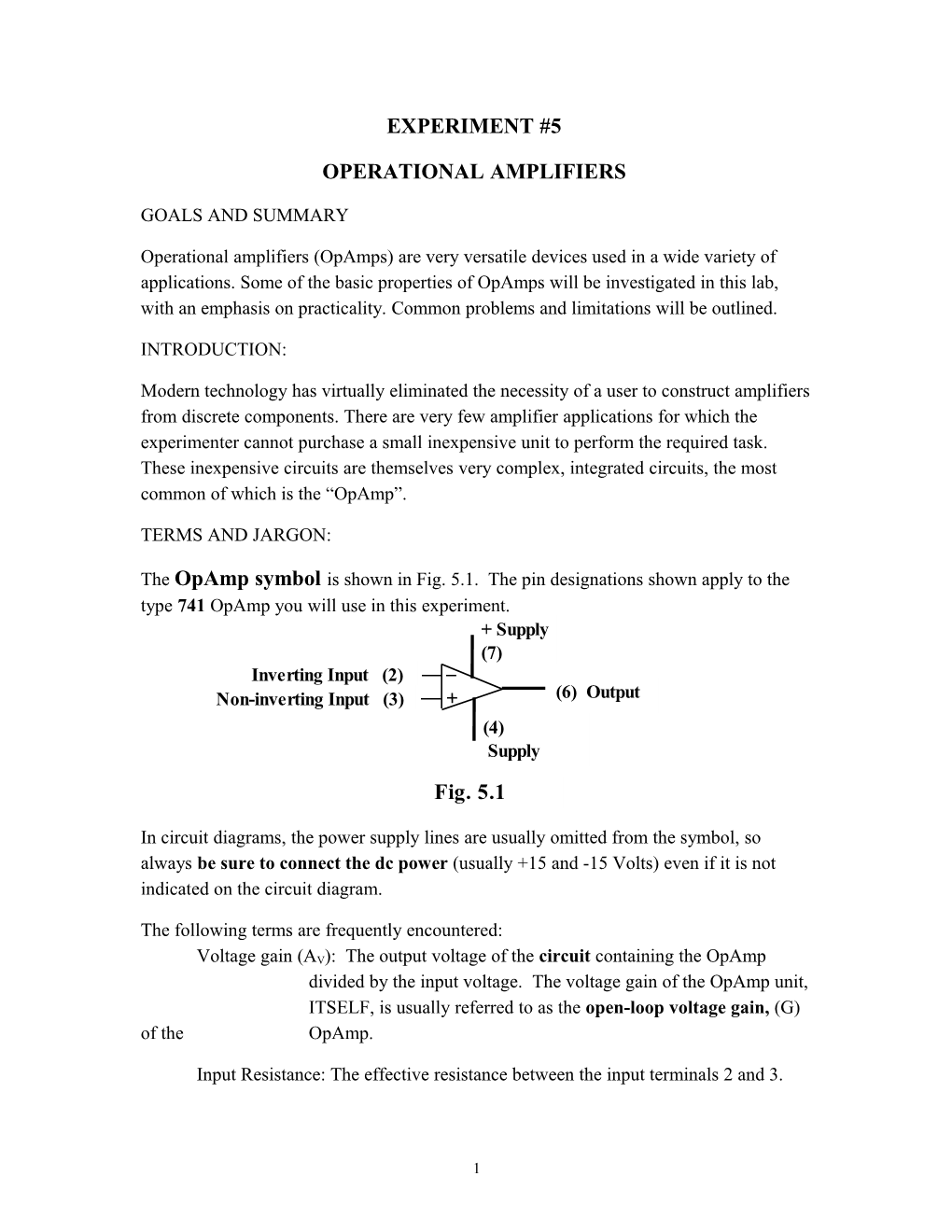# Operational AmplifiersEXPERIMENT #5

OPERATIONAL AMPLIFIERS

GOALS AND SUMMARY

Operational amplifiers (OpAmps) are very versatile devices used in a wide variety of applications. Some of the basic properties of OpAmps will be investigated in this lab, with an emphasis on practicality. Common problems and limitations will be outlined.

INTRODUCTION:

Modern technology has virtually eliminated the necessity of a user to construct amplifiers from discrete components. There are very few amplifier applications for which the experimenter cannot purchase a small inexpensive unit to perform the required task. These inexpensive circuits are themselves very complex, integrated circuits, the most common of which is the “OpAmp”.

TERMS AND JARGON:The OpAmp symbol is shown in Fig. 5.1. The pin designations shown apply to the type 741 OpAmp you will use in this experiment.
In circuit diagrams, the power supply lines are usually omitted from the symbol, so always be sure to connect the dc power (usually +15 and -15 Volts) even if it is not indicated on the circuit diagram.

The following terms are frequently encountered:
Voltage gain (AV): The output voltage of the circuit containing the OpAmp divided by the input voltage. The voltage gain of the OpAmp unit, ITSELF, is usually referred to as the open-loop voltage gain, (G) of the OpAmp.

Input Resistance: The effective resistance between the input terminals 2 and 3.

Output Resistance: The effective resistance in series with the output terminal.

Bandwidth: The frequency range over which the gain is nearly constant.

THE NON-IDEAL CHARACTERISTICS OF OPAMPS TO BE AWARE OF:

1. The amplifier draws a minute amount of current (100 nA) through its inputs at all times. This is called the “input offset current”. The amplifier is also characterised by a slight, D.C. “input offset voltage” (1 mV).
2. The gain of the amplifier decreases with increasing frequency. This is a deliberate design characteristic, which reduces the tendency of the amplifier to oscillate.
3. The value of the output voltage signal can never exceed the power supply voltages.

1.The Inverting Amplifier

A simple but practical amplifier is shown in Fig. 5.2.

Its voltage gain, AV, is given by:

This is the ideal behaviour of the circuit as a linear amplifier (ie. the output voltage is linearly proportional to the input voltage). However, there are several restrictions on this ideal behaviour (and you’ll explore some of these as you do this experiment):

Restrictions:

/ so that input noise, and the input offset current and voltage do not interfere with the measurements.
/ so that the output is not asked to provide voltages greater than is provided by the power supply.
/ Where f is the maximum frequency to be amplified. This gain-bandwidth product is determined by the natural bandwidth and open-loop gain of the OpAmp itself.

Construct the Inverting Amplifier circuit shown in Fig. 5.2 on the circuit board provided, using Ri = 1 k for the input resistor and Rf = 100 k for the feedback resistor. The pin diagrams and details on the 741 OpAmp can be found in you lab bench notes. Use the needle-nose pliers to push the component leads and connecting wires into the appropriate holes in the board. Connections to the signal generator and ’scope should be made via the BNC connectors mounted on the circuit board. You might also connect the second vertical input of the ’scope to a spare connector on the board, which, if connected to a few inch length of wire, will provide you with a convenient “probe” to touch to various points in the circuit when checking circuit operation. In addition, keeping the vertical inputs of the ’scope on d.c. will allow the oscilloscope to be used as a d.c. voltmeter as well as for recording a.c. signals. During your wiring:
Keep the power off until you have checked all connection.

First, use a small amplitude sine wave for the input voltage and try varying the dc offset voltage of the frequency generator. You will find that a dc offset or an input amplitude that is too large can easily cause the amplifier to saturate, giving a distorted or "clipped" waveform. What + and - output voltages does the signal get clipped at? During your work with OpAmps you should always be wary of such distortions and you may find that you need to adjust the dc offset or the amplitude of the generator if the output of the amplifier is saturating.

In addition to this problem of DC offset, there are more subtle distortions that can occur. You can see some of these by increasing the frequency high enough that the output amplitude from the amplifier decreases drastically. Then qualitatively investigate what happens when you vary the amplitude of the input signal. You are likely to see a distorted waveform of some sort and non-linear behaviour (meaning that the output amplitude no longer varies linearly with the input amplitude). These potential problems should be kept in mind when making the remainder of the measurements.

Now, over a wide frequency range, measure the gain AV of the output voltage relative to the input voltage. Determine the bandwidth BW of the amplifier by finding the frequency at which the gain drops to of the gain at low frequencies (always making sure that you are working in a linear regime of the amplifier). Your measurements of the gain should be plotted on a log-log plot and fitted to determine the power law that governs the roll-off above this cutoff frequency.

Next, explore a wide range of gains and bandwidths by using several different combinations of resistors for Ri and Rf. For each case measure the low frequency gain AV and the bandwidth BW. Take sufficient data to clearly determine the relationship between gain and bandwidth for the inverting amplifier. For this latter part, you will need to make quick determinations of the bandwidth by just measuring the low frequency gain and then finding the bandwidth.

1